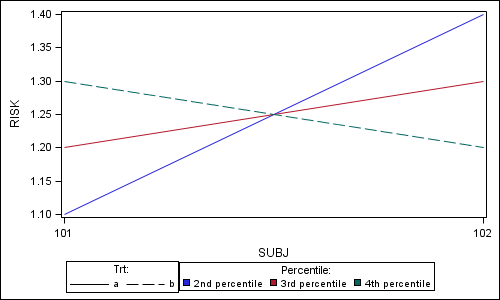## sgplot or sgrender

Hi ,

I need a series plot with xaxis=subject and yaxis=risk with treatment groups a ,b and each subject has again different ht percentiles. so i need to plot 2nd percentile on the graph with red color(dotted pattern will be for trt=a and solid pattern for trt=b) and like wise 3rd percentile on graph with blue color(dotted pattern for trt=a, solid pattern for trt=b) and 4th percentile with orange color(dotted pattern for trt=a , solid pattern for trt=b). and i need to show legends as  red=2nd percentile,blue=3rd percentile,orange=4th percentile,dotted line with no color as trt a, solid line with trt b.

so basically i need to show series plot of different percentile vs trt group.

SUBJ TRT RISK PERCENTILE

101  a       1.1       2nd percentile

101  a       1.2       3rd percentile

101  a       1.3       4th percentile

102  b       1.4       2nd percentile

102  b        1.3       3rd percentile

102  b        1.2       4th percentile

do i need to create 3 variable as var1,var2,var3 for 3 different percentiles because legends are not showing up

proc sgplot data=test;

series xaxis=subj yaxis=var1/ group=trt name='a';

series xaxis=subj yaxis=var2/ group=trt name='b';

series xaxis=subj yaxis=var3/ group=trt name='c';

keylegend 'a' 'b' 'c'

run;

above is sample code. keylegend just has short code.

even if i do this i am not able to create proper legend or do i need to create var1 to var6 (var1 to var3 for trt=a, var4 to var6 for trt=b) and then create colors to line and legend.

thanks,

vk

2 REPLIES 2Jay54
Meteorite | Level 14

## Re: sgplot or sgrender

Yes, you can do this using the LineColorGroup and LinePatternGroup options in the GTL SeriesPlot.  You can plot risk by subject with group=percentile.  However, your data is off a bit, you need trt to be same across a line.  Here is the graph and code:data values;

input SUBJ \$ TRT \$ RISK PERCENTILE \$14-27;

datalines;

101  a  1.1  2nd percentile

101  a  1.2  3rd percentile

101  b  1.3  4th percentile

102  a  1.4  2nd percentile

102  a  1.3  3rd percentile

102  b  1.2  4th percentile

run;

proc template;
define statgraph PowerSeries;
begingraph;
layout lattice;
layout overlay;
seriesplot x=subj y=risk / group=percentile
linecolorgroup=percentile linepatterngroup=trt name='a';
endlayout;
sidebar / align=bottom spacefill=false;
layout gridded / columns=2;
discretelegend 'a' / title='Trt:' type=linepattern across=2;
discretelegend 'a' / title='Percentile:' type=linecolor across=3;
endlayout;
endsidebar;
endlayout;
endgraph;
end;
run;

ods listing;
ods graphics / reset width=5in height=3in imagename='PowerSeries';
proc sgrender data=values template=PowerSeries;
run;

## Re: sgplot or sgrender

Thanks sanjay. This is what i need.

Discussion stats
• 2 replies
• 1474 views
• 0 likes
• 2 in conversation